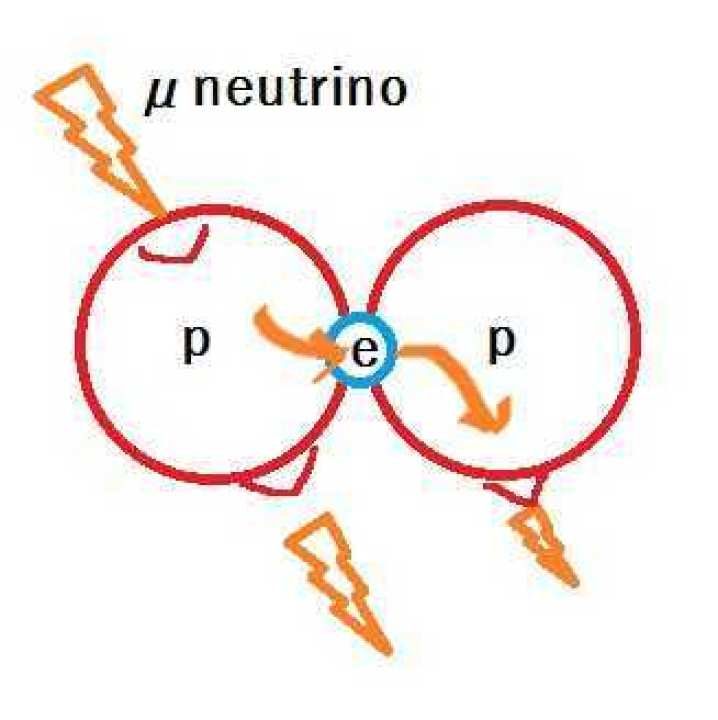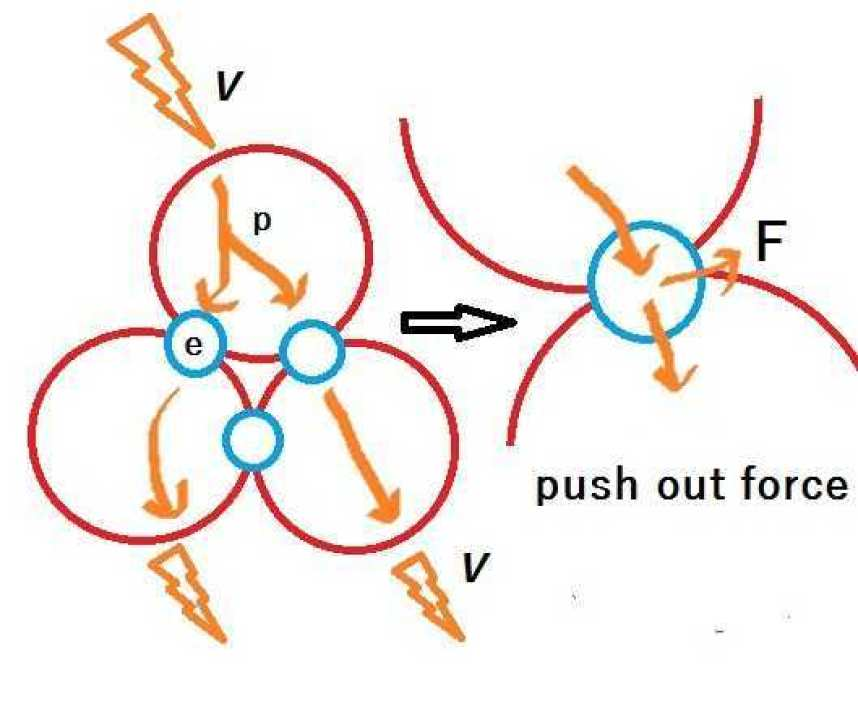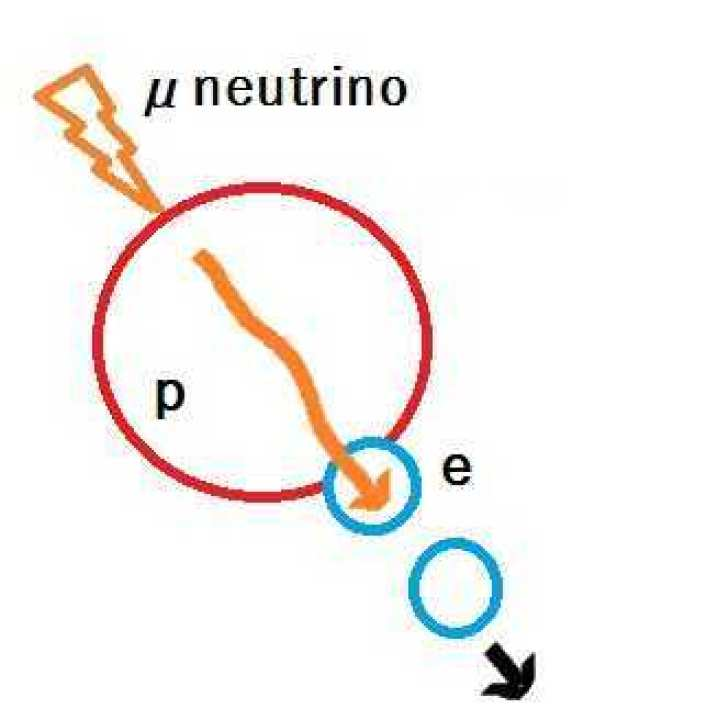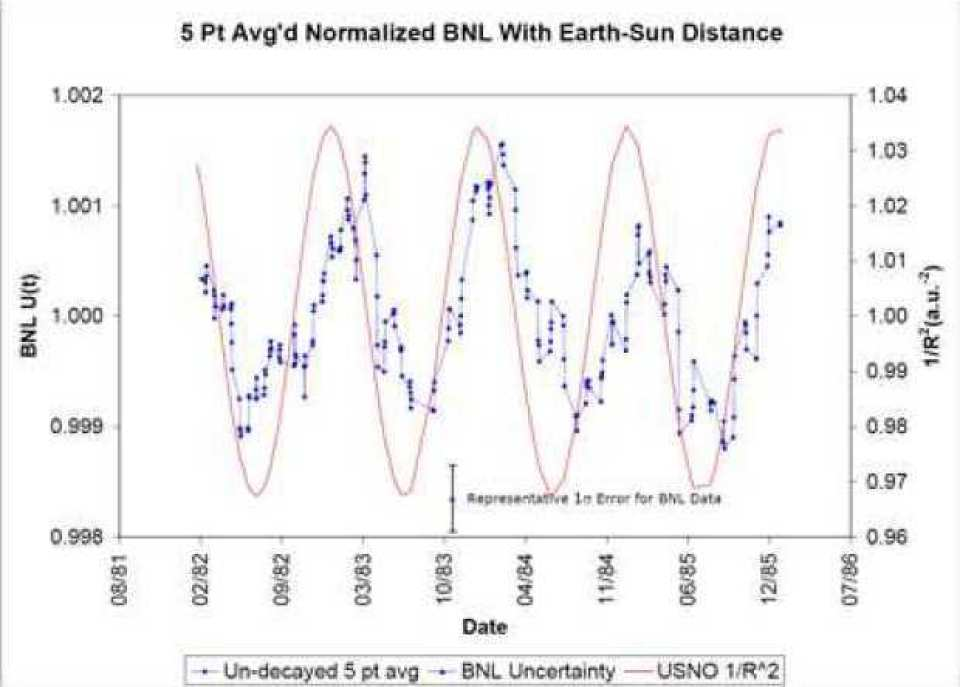# Neutron decay by neutrino

## Neutron decay by neutrino

Article - 4 years 8 months ago

Neutron was assumed to be a combination of protons and electrons. There is a hydrogen atom composed of the same proton and electron. Actual hydrogen atoms are two molecules bound, but the masses of neutrons and hydrogen atoms are like this. I want the effective digits to close their eyes.

Neutron n → 1.674927471 x 10 ^ -27 kg

Hydrogen atom H → 1.6737236 × 10 ^ -27 kg

Even the same proton and electron, the neutron is a bit heavier. The difference,

n - H → 0.001203871 x 10 ^ -27 kg

What is the difference?

By the way, deuterium does not decay with stable isotope.

Deuterium 2H → 3.343583719 x 10 ^ -27 kg

We subtract the weight of one orbital electron and two protons from deuterium.

2H - e - 2p -> 0.000749 x 10 ^ -27 kg

The weight of electrons,

Electron e → 0.00091093 x 10 ^ -27 kg

Almost one electron weight comes out. Is this the weight of electrons linking protons and protons?

So I compared the weight of tritium and three protons.

Tritium 3H → 5.00827094 x 10 ^ -27 kg

Subtract the weight of one orbital electron.

3H + → 5.00736 × 10 ^ -27 kg

Three protons

3xp → 5.017865484 x 10 ^ -27 kg

??? Three protons are heavier!

3xp - 3H + → 0.010505484 x 10 ^ -27kg

It is thought that tritium is bonded with three protons and three electrons. So what is the weight of helium 3?

3He → 5.00823789 x 10 ^ -27 kg

3He - 2 e -> 5.006416 x 10 - -27 kg

3H + - 3He ++ -> 0.000944 x 10 ^ -27 kg

Tritium has three protons with three electrons, helium 3 with three protons coupled with two electrons. The difference is just one electron. This is in line with the nuclear model expected in Electrical Earth Sciences. Tritium decays in beta, but helium 3 is a stable isotope. The difference in structure is that tritium is triangle and helium 3 is straight.

Here I will make a fairly leap forward.Neutrino passes through the nucleus of deuterium

Deuterium binds two protons with electrons. When neutrino radiation is received, an electric field is transmitted inside the protons. The electric field of the neutrino also moves to the next proton via the combined electrons. At this time, the pulses of the electric field passing through the electrons are straight. Three protons are also connected in series in helium 3.Helium 3

Electrons connecting protons and protons are bounced off by neutrino

Even with tritium hydrogen, the pulse of the electric field of the neutrinos is transmitted inside the protons, but the electrons linking the adjacent protons are angled. For this reason, a force toward the outside is generated inside the electrons. If neutrinos with very high energy are incident, they will skip the electrons outward. This is beta decay.

It is clear why neutrons will decay so far.hit by neutorino

A neutrino incident on a proton will eject electrons. The ejected electron gains energy of neutrino and fly away. Since the protons are slightly deformed, an electron neutrino also occurs.

Still, there is a doubt why 3H+ <3xp in this consideration, but there is no contradiction in the action of re-generation of electric field and supply of electric charge by neutrino.

Since there was a paper supporting the prediction that neutrinos are working for beta decay, I will introduce it.Evidence for Correlations Between Nuclear Decay Rates and Earth-Sun Distance

Evidence for Correlations Between Nuclear Decay Rates and Earth-Sun Distance

This graph is evidence that the change in the distance between the sun and the earth has a strong correlation with the half-life of 36Cl-32Si. The blue dot is the half-life, the red line is the distance between the earth and the sun. 36 Cl is caused by collision of cosmic rays from argon in the atmosphere. Beta decay with a half-life of about 300,000 years - It changes to 32 Si with alpha decay. Precise examination of this half life corresponds to the change in the distance between the earth and the sun. There is speculation that the solar neutrinos may work in some way. When the distance to the sun is short, the half-life becomes short. It is because the concentration of neutrinos increases.